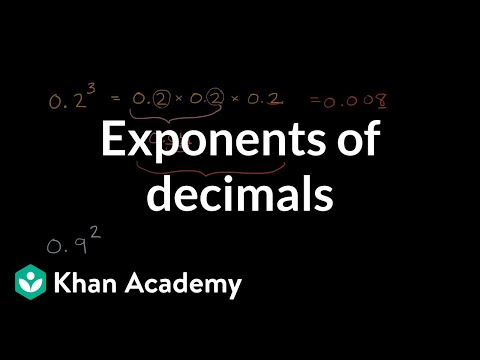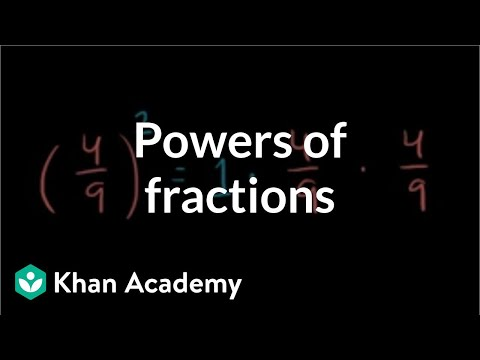Video

# Intro to exponents (Full video)

Description: Sal shows how exponents are repeated multiplication. Created by Sal Khan. So, if we had 2 times 3 (2 × 3), we could literally view this as 3 2's being added together. What we're going to introduce you to in this video is the idea of repeated multiplication – a new operation that really can be viewed as repeated multiplication. So now, let's take the idea of 2 to the 3rd power (2^3) – which is how we would say this.

### Other videos you might be interested in### Exponents of decimals (Full video)